Courses

# Flow Dynamics in Open Channels and Rivers (Part - 2) Civil Engineering (CE) Notes | EduRev

## Civil Engineering (CE) : Flow Dynamics in Open Channels and Rivers (Part - 2) Civil Engineering (CE) Notes | EduRev

The document Flow Dynamics in Open Channels and Rivers (Part - 2) Civil Engineering (CE) Notes | EduRev is a part of Civil Engineering (CE) category.
All you need of Civil Engineering (CE) at this link: Civil Engineering (CE)

Uniform flow in channels of simple cross section

For problems concerning the steady uniform flow in rivers and open channels, the Manning’s equation is commonly used in India. The depth of water corresponding to a discharge in a channel or river under uniform flow conditions is called “normal depth”. By combining the continuity equation with that of Mannings, one obtains

Q = 1/n AR 2/3 S1/2                   (12)

Where the variables have been defined in the earlier sections.

One may also write equation (12) as follows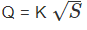Where K =  AR 2/3/n ,also called Conveyance, is often necessary to find out the normal depth of flow corresponding to a discharge Q, flowing in a channel for which equation (11) may be rearranged as

AR 2/3 = nQ/S1/2

In equation (14), the right hand side terms are known where as those in left hand are unknown and are functions of water depth. For a few commonly encountered sections the parameters A and R are given in the table below.

 Rectangle Trapezoid Circle Flow Area, A b.h (b+my).y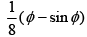Wetted Perimeter, P b +2h b +2h.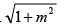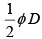Hydraulic Radius, R bh/b + 2h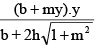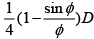Free surface width, B b b +2mh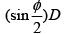In the table, m stands for the side slope of a trapezoidal channel and  stands for the angle subtended at the centre by the water surface chord line.

As seen from the above table except for the very simple rectangular section it is not possible directly to evaluate h, corresponding to Q as the left hand side of equation 13 is nonlinear in terms of h. One way of solving is by Newton’s method, where equation (14) is written as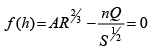(15)

For using Newton’s method the derivative of the function is required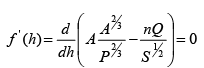(16)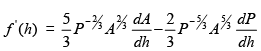(17)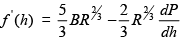(18)

Where we have used dA/dh = B Similarly the expression dP/dh may be evaluated for  any section.

Starting with a realistic value hthe iteration may be carried out as given below: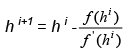(19)

Where h i+1 is the value of h at next iteration, which is an improvement of initial guess hi. The iteration may be continued till a desired accuracy is achieved.

Uniform flow in channels of compound cross section

A compound section may be defined as a section in which various portions of the cross-section have different flow properties, like surface roughness or channel depth. (Figure 15)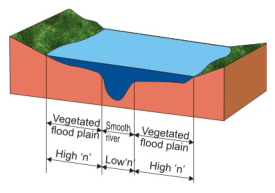FIGURE 15. A river in flood example of a compound flow section

In order to use the uniform flow formula in compound channels one way may be to divide the flow section into sub areas (Figure 16) and treat the flow in each area separately.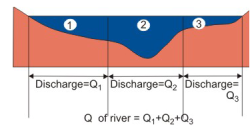FIGURE 16. Simple flow analysis method for compound channels

However, it has been found that this method may lead to errors by as much as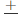20 or even more (Chadwick et al 2004). The error is largely due to the neglecting of mass and momentum interchange between adjacent sub-areas. The current solution would however be more complex by using a two or even three-dimensional model.

In another method, the energy coefficient (α) and friction slope Sf are evaluated in terms of conveyance K of the sub areas. With these expressions, the flow in compound section may be computed without knowing the individual flows in each sub area. For a compound channel divided into N sections. (For example N = 3 in Figure 15). The energy coefficient,α, is found out as: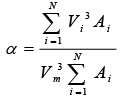(20)

Where Vm is the mean flow velocity in the entire section and is given as follows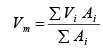(21)

Where Vi = Qi /Ai and Ai is the area of its ith sub-area. Equation (18) now can be written as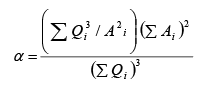(22)

Now, the flow in sub-areas i may be written as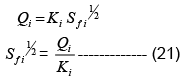(23)

Here, an assumption has been made that Sf has the same value for all subareas, which is not quite correct since the velocities of each of these areas being different, would not give equal velocity heads. Where as, the water surface is almost level over the entire cross section.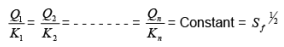(24)

It follows from equation (23) that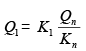(25)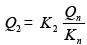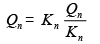(26)

Adding all the above equation yields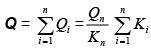(27)

By substituting this expression for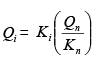into equation (27) and simplifying  the equation, one obtains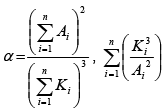(28)

Elimination of  Qn/Kn from equations (24) and (26) and squaring both sides give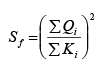(29)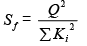(30)

Thus, expressions for α and Shave been evaluated for any given stage without explicitly determining the flow in each sub areas, Qi. In addition, equation (30) may be used in the procedure for determining varied flow profiles as discussed .

Non uniform in channels

There are quite a few examples of non-uniform flow in rivers or open channels that may be encountered by a water resources engineer. Some of these have been illustrated in Figure 17.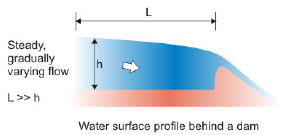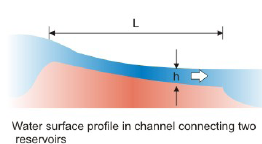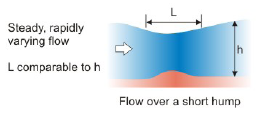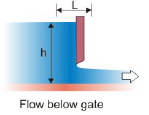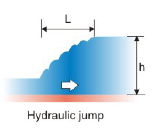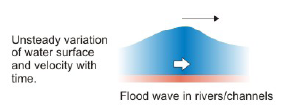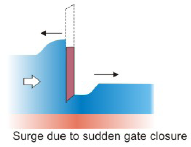FIGURE 17. Some example of flow situation encountered in practice.

In this lesson we shall discuss the procedure to evaluate water surface profiles for steady, gradually varying flow situations. For steady, rapidly varying and unsteady flow situations, reference may be made to following or similar texts on hydraulics of open channel flow, like Ranga Raju (2003) or Subramanya (2002).

A representative non-uniform gradually varied flow is shown in Figure 18.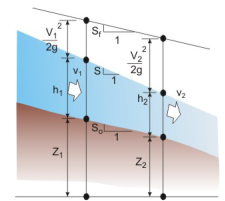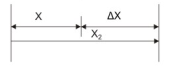Figure 18. Non-uniform gradually varied flow

Over the incremental distance Δx, the depth and velocity are known to change slowly. The slope of the energy grade line is designated as α in contrast to uniform flow, the slopes of the energy grade line, water surface, and channel bottom are no longer parallel. Since the changes in the water depth h and velocity V are gradual, the energy lost over the incremental Δx can be represented by manning equation. This means that equation 11, which is valid for uniform flow can also be used to evaluate S for a gradual varied flow situation, and that the roughness coefficients discussed in Section  are applicable.

Additional assumption includes a regular cross section, small channel slope, hydrostatic pressure distribution and one-dimensional flow.

Applying the equivalence of energy between locations 1 and 2, and assuming the loss term as hL given by Sf.Δx one obtains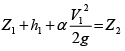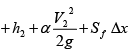(31)

In the above equation, Δx is the distance between two consecutive sections x1 and x2 such that Δx=x2- x1.

The energy coefficient α has been used along with the V2/2g  term, as it may be much different from 1.0 for natural sections. The term sf in equation (31) may be  evaluated by the expression for uniform flow, equation (11), where S0 may be replaced by sf .Since equation (31) relates the energy between the sections, Sf may be taken either of the following:

Arithmetic mean: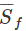= 1/2 (Sf1 + Sf2)               (32)

Geometric mean:=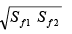(33)

Harmonic mean:=(34)

Where Sf1 and Sf2 are the friction slopes evaluated at section 1 and 2 by using the Mannings formula equation (12).

Equation (29) may be used by starting from one end of the channel where the flow depth and velocity are known and working backward or forward in steps. Here, two, methods are used of which we shall discuss one, called the standard step method. Avery popular computer program called HEC-2 developed by hydrologic engineering center of the US Army Corps of Engineers is based on this method.

In the standard step method, for any given discharge the depth of flow would be known at the control section. It is then required to calculate the depth of flow at the section immediately next to the control section. Two examples are illustrated in Figure 19.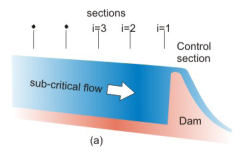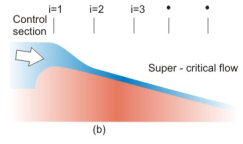Figure 19. Computation grid by the standard step method.
(a)  profile behind a dam; calculation proceeds upstream from control section
(b)  profile in a steep channel ; calculation proceeds downstream from control section.

The distance between the two successive sections (i and i+1) is taken as constant, say Δx. It may be observed from the Figure 19a since the water is flowing above the dam the water depth above the dam crest can be found out for the given discharge. Hence the water level at the control section just upstream of the dam is known. Similarly, in Figure 19b, since the water is flowing down from the reservoir into the steep channel critical depth corresponding to the given discharge would exist at the control section. Here two, the water level at the control section is then known.

Starting at the control section (i =1), the total energy of water is found out to be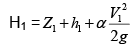(35)

Next, consider the first reach, that is, between sections i =1 and i =2. A depth of flow is assumed at section 2 and the energy there, that is,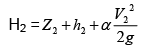(36)

is evaluated. Now, one of the equations for finding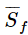(the average friction slope) in the reach is found out by, say equation 31.

As may be observed from Figure 18 the numerical value of H2 found from equation (33) should be equal to that of h1 found from equation (33) + Sf. If the depth at the section 2 has been correctly assumed if the two don’t match, a new  depth h2 is assumed and the calculations are repeated till the two values match.

Once a correct depth is found at section 2, a similar procedure is used to find the depth at section 3, and so on.

Offer running on EduRev: Apply code STAYHOME200 to get INR 200 off on our premium plan EduRev Infinity!

,

,

,

,

,

,

,

,

,

,

,

,

,

,

,

,

,

,

,

,

,

;